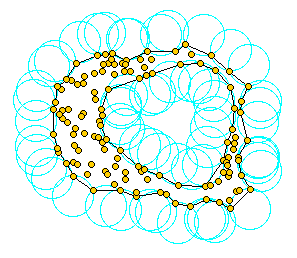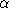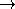# Chapter 292D Alpha Shapes

Tran Kai Frank Da

 29.1 Definitions 29.2 Functionality 29.3 Concepts and Models 29.4 Examples 29.4.1   Example for Basic Alpha-Shapes 29.4.2   Example for Basic Alpha-Shapes with Many Points 29.4.3   Example for Weighted Alpha-ShapesAssume we are given a set S of points in 2D or 3D and we'd like to have something like ``the shape formed by these points.'' This is quite a vague notion and there are probably many possible interpretations, the-shape being one of them. Alpha shapes can be used for shape reconstruction from a dense unorganized set of data points. Indeed, an-shape is demarcated by a frontier, which is a linear approximation of the original shape [BB97].

As mentioned in Edelsbrunner's and Mücke's paper [EM94], one can intuitively think of an-shape as the following. Imagine a huge mass of ice-cream making up the space3 and containing the points as ``hard'' chocolate pieces. Using one of these sphere-formed ice-cream spoons we carve out all parts of the ice-cream block we can reach without bumping into chocolate pieces, thereby even carving out holes in the inside (e.g. parts not reachable by simply moving the spoon from the outside). We will eventually end up with a (not necessarily convex) object bounded by caps, arcs and points. If we now straighten all ``round'' faces to triangles and line segments, we have an intuitive description of what is called the-shape of S. Here's an example for this process in 2D (where our ice-cream spoon is simply a circle):

And what isin the game?is the squared radius of the carving spoon. A very small value will allow us to eat up all of the ice-cream except the chocolate points themselves. Thus we already see that the-shape degenerates to the point-set S for0. On the other hand, a huge value ofwill prevent us even from moving the spoon between two points since it's way too large. So we will never spoon up ice-cream lying in the inside of the convex hull of S, and hence the-shape foris the convex hull of S.1

## 29.1   Definitions

We distinguish two versions of alpha shapes. Basic alpha shapes are based on the Delaunay triangulation. Weighted alpha shapes are based on its generalization, the regular triangulation, replacing the euclidean distance by the power to weighted points.

There is a close connection between alpha shapes and the underlying triangulations. More precisely, the-complex of S is a subcomplex of this triangulation of S, containing the-exposed k-simplices, 0kd. A simplex is-exposed, if there is an open disk (resp. ball) of radius sqrt() through the vertices of the simplex that does not contain any other point of S, for the metric used in the computation of the underlying triangulation. The corresponding-shape is defined as the underlying interior space of the-complex (see [EM94]).

In general, an-complex is a non-connected and non-pure polytope, it means, that one k-simplex, 0kd-1 is not necessary adjacent to a (k+1)-simplex.

The-shapes of S form a discrete family, even though they are defined for all real numberswith 0. Thus, we can represent the entire family of-shapes of S by the underlying triangulation of S. In this representation each k-simplex of the underlying triangulation is associated with an interval that specifies for which values ofthe k-simplex belongs to the-shape. Relying on this fact, the family of-shapes can be computed efficiently and relatively easily. Furthermore, we can select an appropriate-shape from a finite number of different-shapes and corresponding-values.

## 29.2   Functionality

The class CGAL::Alpha_shape_2<Dt> represents the family of-shapes of points in a plane for all positive. It maintains the underlying triangulation Dt which represents connectivity and order among squared radius of its faces. Each k-dimensional face of the Dt is associated with an interval that specifies for which values ofthe face belongs to the-shape. There are links between the intervals and the k-dimensional faces of the triangulation.

The class CGAL::Alpha_shape_2<Dt> provides functions to set and get the current-value, as well as an iterator that enumerates the-values where the-shape changes.

It provides iterators to enumerate the vertices and edges that are in the-shape, and functions that allow to classify vertices, edges and faces with respect to the-shape. They can be in the interior of a face that belongs or does not belong to the-shape. They can be singular/regular, that is be on the boundary of the-shape, but not incident/incident to a triangle of the-complex.

Finally, it provides a function to determine the-value such that the-shape satisfies the following two properties, or at least the second one if there is no suchthat both are satisfied:

(i) The number of components equals a number of your choice and
(ii) all data points are either on the boundary or in the interior of the regularized version of the-shape (no singular edges).

The current implementation is static, that is after its construction points cannot be inserted or removed.

## 29.3   Concepts and Models

We currently do not specify concepts for the underlying triangulation type. Models that work for a basic alpha-shape are the classes CGAL::Delaunay_triangulation_2 and CGAL::Triangulation_hierarchy_2 templated with a Delaunay triangulation. A model that works for a weighted alpha-shape is the class CGAL::Regular_triangulation_2.

The triangulation needs a geometric traits class as argument. The requirements of this class are described in the concept CGAL::AlphaShapeTraits_2 for which the CGAL kernels and CGAL::Weighted_alpha_shape_euclidean_traits_2 are models.

There are no requirements on the triangulation data structure. However it must be parameterized with vertex and face classes, which are model of the concepts AlphaShapeVertex_2 and AlphaShapeFace_2, by default the classes CGAL::Alpha_shape_vertex_base_2<Gt> and CGAL::Alpha_shape_face_base_2<Tf>.

## 29.4   Examples

### 29.4.1   Example for Basic Alpha-Shapes

The basic alpha shape needs a Delaunay triangulation as underlying triangulation Dt. The Delaunay triangulation class is parameterized with a geometric and a triangulation data structure traits.

For the geometric traits class we can use a CGAL kernel.

For the triangulation data structure traits, we have to choose the vertex and face classes needed for alpha shapes, namely CGAL::Alpha_shape_vertex_base_2<Gt, Dv> and CGAL::Alpha_shape_face_base_2<Gt,Df>. As default vertex and face type they use CGAL::Triangulation_vertex_base_2<Gt> and CGAL::Triangulation_face_base_2<Gt> respectively.

The following code snippet shows how to obtain a basic alpha shape type.

```typedef CGAL::Cartesian<double> K;

typedef CGAL::Alpha_shape_vertex_base_2<K> Av;

typedef CGAL::Triangulation_face_base_2<K> Tf;
typedef CGAL::Alpha_shape_face_base_2<K,Tf> Af;

typedef CGAL::Triangulation_default_data_structure_2<K,Av,Af> Tds;
typedef CGAL::Delaunay_triangulation_2<K,Tds> Dt;
typedef CGAL::Alpha_shape_2<Dt> Alpha_shape_2;
```

### 29.4.2   Example for Basic Alpha-Shapes with Many Points

When the input data set is huge, say more than 10.000 points, it pays off to use a triangulation hierarchy. It has the same API as the Delaunay triangulation and differs only in the types of the vertices and faces. Therefore, the only part that changes are the typedefs in the beginning.

```typedef CGAL::Cartesian<double> K;

typedef CGAL::Alpha_shape_vertex_base_2<K> Avb;
typedef CGAL::Triangulation_hierarchy_vertex_base_2<Avb> Av

typedef CGAL::Triangulation_face_base_2<K> Tf;
typedef CGAL::Alpha_shape_face_base_2<K,Tf> Af;

typedef CGAL::Triangulation_default_data_structure_2<K,Av,Af> Tds;
typedef CGAL::Delaunay_triangulation_2<K,Tds> Dt;
typedef CGAL::Triangulation_hierarchy_2<Dt> Ht;
typedef CGAL::Alpha_shape_2<Ht> Alpha_shape_2;
```

### 29.4.3   Example for Weighted Alpha-Shapes

A weighted alpha shape , needs a regular triangulation as underlying triangulation Dt, and it needs a particular face class, namely CGAL::Regular_triangulation_face_base_2<Gt>. Note that there is no special weighted alpha shape class.

```typedef CGAL::Cartesian<double> K;
typedef CGAL::Weighted_alpha_shape_euclidean_traits_2<K> Gt;

typedef CGAL::Alpha_shape_vertex_base_2<Gt> Av;

typedef CGAL::Regular_triangulation_face_base_2<Gt> Rf;
typedef CGAL::Alpha_shape_face_base_2<Gt,Rf>  Af;

typedef CGAL::Triangulation_default_data_structure_2<Gt,Av,Af> Tds;
typedef CGAL::Regular_triangulation_2<Gt,Tds> Rt;
typedef CGAL::Alpha_shape_2<Rt> Alpha_shape_2;
```

### Footnotes

 1 ice cream, ice cream!!! The wording of this introductory paragraphs is borrowed from Kaspar Fischer's `` Introduction to Alpha Shapes'' which can be found at http://n.ethz.ch/student/fischerk/alphashapes/as/index.html. The picture has been taken from Walter Luh's homepage at http://www.stanford.edu/&wtilde;luh/cs448b/alphashapes.html.